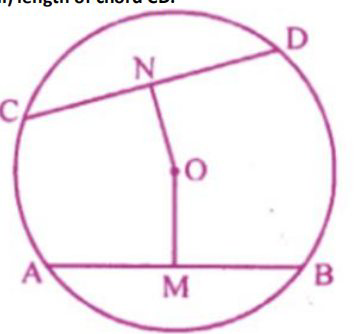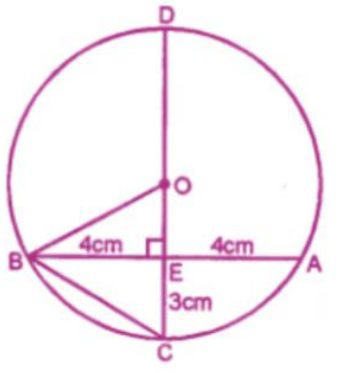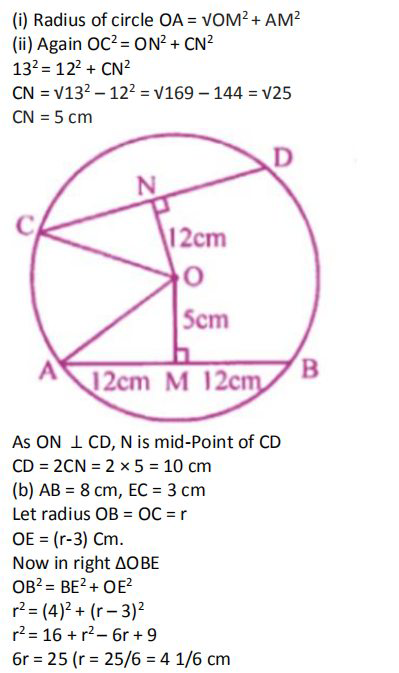# ML Aggarwal Solutions Class 9 Mathematics Solutions for Circle Exercise 15.1 in Chapter 15 - Circle

(a) In the figure given below, O is the center of the circle. AB and CD are two chords of the circle, OM is perpendicular to AB and ON is

perpendicular to CD. AB = 24 cm,

OM = 5 cm, ON = 12 cm. Find the:

(i) the radius of the circle.

(ii) length of chord CD.(b) In the figure (ii) given below, the CD is the diameter which meets the chord AB in E

such that AE = BE = 4 cm. If CE = 3 cm, find the radius of the circle.that

(a)Given : AB = 24 cm, OM = 5cm, ON = 12cm

OM ⊥ AB

M is the midpoint of AB

AM = 12 cmRelated Questions

Lido

Courses

Teachers

Book a Demo with us

Syllabus

Maths
CBSE
Maths
ICSE
Science
CBSE

Science
ICSE
English
CBSE
English
ICSE
Coding

Terms & Policies

Selina Question Bank

Maths
Physics
Biology

Allied Question Bank

Chemistry
Connect with us on social media!